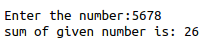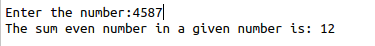• #5, First Floor, 4th Street Dr. Subbarayan Nagar Kodambakkam, Chennai-600 024 Landmark : Samiyar Madam
• pro@slogix.in
• +91- 81240 01111

### What is Looping in python?

###### Description

To see the kinds of looping in python3.

#### If loop.

It checks the condition is true, then its execute that particular block of code.

#### For loop.

It executes a sequence of statements multiple times until the condition goes False.

#### While loop.

Repeats a statement while a given condition is True.

It tests the condition before executing the loop body.

###### Sample Code

Source code(While loop):

#get input from user

n=int(input(“Enter the number:”))

rev=0

#initiate while loop

while(n>0):

r=n%10

rev=rev+r

n=n//10

#print the result

print(“sum of given number is:”,rev)

Source code(for and if loop):

#get input from user

n=input(“Enter the number:”)

total=0

#initialize for loop

for i in n:

#Type casting

(because we cant take integer input in loop)

a=int(i)

#initialize if loop

if(a%2==0):

total=total+a

#print the result

print(“The sum even number in a

given number is:”,total)

###### Screenshots